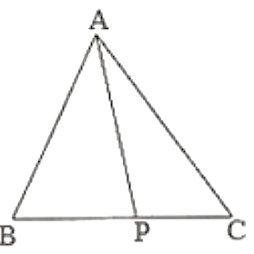×#### Thank you for registering.

One of our academic counsellors will contact you within 1 working day.

Click to Chat

1800-1023-196

+91-120-4616500

CART 0

• 0

MY CART (5)

Use Coupon: CART20 and get 20% off on all online Study Material

ITEM
DETAILS
MRP
DISCOUNT
FINAL PRICE
Total Price: Rs.

There are no items in this cart.
Continue Shopping• Complete JEE Main/Advanced Course and Test Series
• OFFERED PRICE: Rs. 15,900
• View Details

```Chapter 15: Properties of Triangles Exercise – 15.4

Question: 1

In each of the following, there are three positive numbers. State if these numbers could possibly be the lengths of the sides of a triangle:

(i) 5, 7, 9

(ii) 2, 10.15

(iii) 3, 4, 5

(iv) 2, 5, 7

(v) 5, 8, 20

Solution:

(i) Yes, these numbers can be the lengths of the sides of a triangle because the sum of any two sides of a triangle is always greater than the third side. Here, 5 + 7 > 9, 5 + 9 > 7, 9 + 7 > 5

(ii) No, these numbers cannot be the lengths of the sides of a triangle because the sum of any two sides of a triangle is always greater than the third side, which is not true in this case.

(iii) Yes, these numbers can be the lengths of the sides of a triangle because the sum of any two sides of triangle is always greater than the third side. Here, 3 + 4 > 5, 3 + 5 > 4, 4 + 5 > 3

(iv) No, these numbers cannot be the lengths of the sides of a triangle because the sum of any two sides of a triangle is always greater than the third side, which is not true in this case. Here, 2 + 5 = 7

(v) No, these numbers cannot be the lengths of the sides of a triangle because the sum of any two sides of a triangle is always greater than the third side, which is not true in this case. Here, 5 + 8 < 20

Question: 2

In Fig, P is the point on the side BC. Complete each of the following statements using symbol ‘=’,’ > ‘or ‘< ‘so as to make it true:

(i) AP… AB+ BP

(ii) AP… AC + PC

(iii) AP…. 1/2(AB + AC + BC)Solution:

(i) In triangle APB, AP < AB + BP because the sum of any two sides of a triangle is greater than the third side.

(ii) In triangle APC, AP < AC + PC because the sum of any two sides of a triangle is greater than the third side.

(iii) AP < 12(AB + AC + BC) In triangles ABP and ACP, we can see that:

AP < AB + BP…(i) (Because the sum of any two sides of a triangle is greater than the third side)

AP < AC + PC…(ii) (Because the sum of any two sides of a triangle is greater than the third side)

On adding (i) and (ii), we have:

AP + AP < AB + BP + AC + PC

2AP < AB + AC + BC (BC = BP + PC)

AP < (AB – FAC + BC)

Question: 3

P is a point in the interior of △ABC as shown in Fig. State which of the following statements are true (T) or false (F):

(i) AP + PB < AB

(ii) AP + PC > AC

(iii) BP + PC = BC

Solution:

(i) False

We know that the sum of any two sides of a triangle is greater than the third side: it is not true for the given triangle.

(ii) True

We know that the sum of any two sides of a triangle is greater than the third side: it is true for the given triangle.

(iii) False

We know that the sum of any two sides of a triangle is greater than the third side: it is not true for the given triangle.

Question: 4

O is a point in the exterior of △ABC. What symbol ‘>’,’<’ or ‘=’ will you see to complete the statement OA+OB….AB? Write two other similar statements and show that

OA + OB + OC > 1/2(AB + BC +CA)

Solution:

Because the sum of any two sides of a triangle is always greater than the third side, in triangle OAB, we have:

OA + OB > AB — (i)

OB + OC > BC — (ii)

OA + OC > CA — (iii)

On adding equations (i), (ii) and (iii) we get:

OA + OB + OB + OC + OA + OC > AB + BC + CA

2(OA + OB + OC) > AB + BC + CA

OA + OB + OC > (AB + BC + CA)/2

Question: 5

In ∆ABC, ∠B = 30°, ∠C = 50°. Name the smallest and the largest sides of the triangle.

Solution:

Because the smallest side is always opposite to the smallest angle, which in this case is 30°, it is AC. Also, because the largest side is always opposite to the largest angle, which in this case is 100°, it is BC.
```### Course Features

• 728 Video Lectures
• Revision Notes
• Previous Year Papers
• Mind Map
• Study Planner
• NCERT Solutions
• Discussion Forum
• Test paper with Video Solution